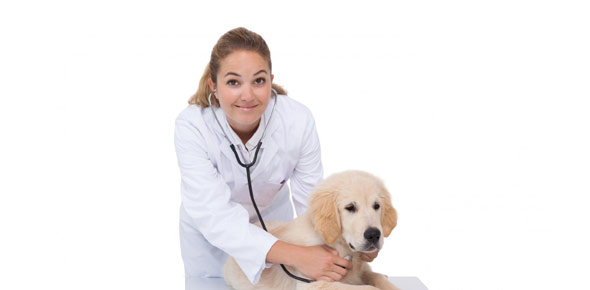# Vet Science Volume Quiz

12 Questions | Total Attempts: 276SettingsVeterinary science is the study of the diagnosis, treatment and medication of animals. Are you a Vet or training to be one? Have you well mastered this science? The quiz below gauges how much and how good you are.

• 1.
How many pints are in a quart?
• A.

1 1

• B.

2

• C.

3

• D.

4

• 2.
How many cups make a pint?
• A.

1

• B.

2

• C.

3

• D.

4

• 3.
1/2 cup is equal to 6 fl oz.
• A.

True

• B.

False

• 4.
Quantricide is prepared by added 2 oz to one gallon of water. How much Quantricide would be used to prepare 1 quart of solution?
• A.

1/2oz

• B.

1oz

• C.

1.5oz

• D.

2.0oz

• 5.
A Technician determines that she has 1 gallon of oatmeal shampoo, 2 quarts of flea shampoo, and 12 cups of green apple conditioner. What is the total number of shampoo and conditioner in pints?
• A.

10pints

• B.

14 pints

• C.

16 pints

• D.

18 pints

• 6.
1 cup is equal to?
• A.

100ml

• B.

150ml

• C.

200ml

• D.

250ml

• 7.
4 quarts are in 1 gallon.
• A.

True

• B.

False

• 8.
A sick lion at the zoo drinks 4 quarts of water, 3 cups of broth, 16 tablespoons of honey, 1 pint of milk, and 16 oz of antibioitc. What was his total fluid intake for the day in fluid ounces?
• A.

180oz

• B.

182 oz

• C.

184oz

• D.

186oz

• 9.
A solution to kill parvovirus germs is mixed 1 cup of Clorox with 1 quart of water. If 2.5 gallons of water is used, how much clorox needs to be added?
• A.

6 cups

• B.

8 cups

• C.

10 cups

• D.

12 cups

• 10.
Hailey and Brynn.. this one is for you. A guinea pig cage water bottle holds 6 oz of water. How many ml are in the bottle?
• 11.
A lamb is receiving 2 tsp of penicillin orally every 6 hours. How many ml of penicillin will the lamb receive in 24 hours?
• 12.
1 tsp is equal to how many ml ?
Related TopicsBack to top